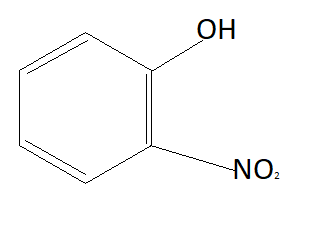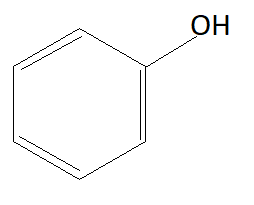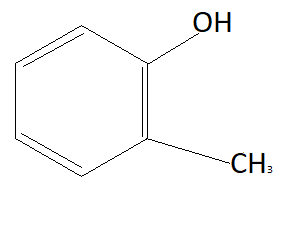QuestionAnswers

# Which of the following compounds is most acidic?A: $Cl - C{H_2} - C{H_2} - OH$ B:C:D:As we know phenols are more acidic than alcohols because of phenoxide ions. When phenols lose their hydrogen phenoxide ion is formed which is highly stable due to which phenols readily lose their hydrogen atom and form phenoxide ion. Chemical formula of phenoxide ion is ${C_6}{H_5}{O^ - }$. Among given options option A is alcohol and rest of the options are phenols. This means option A is not the answer.
Now the option will be eliminated on the basis of the electron withdrawing group. Alkyl group that is $C{H_3}$ is an electron donating group. This means it will donate electrons to the benzene ring and reduce the chances to pull electrons from hydrogen. This means it is increasing the bond strength of $O - H$ which reduces the possibility of losing hydrogen ions. This means option D is also not the answer (as it contains an electron donating group). $N{O_2}$ is an electron withdrawing group that means it will pull electrons from the benzene ring and increases the possibility of taking electrons from hydrogen atoms. Therefore phenol having $N{O_2}$ group is more acidic than phenol. So correct answer is option B that is: